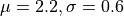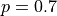# Create and draw scalar distributions¶

import openturns as ot
import openturns.viewer as viewer
from matplotlib import pylab as plt

ot.Log.Show(ot.Log.NONE)


## A continuous distribution¶

We build a normal distribution with parameters :distribution = ot.Normal(2.2, 0.6)
print(distribution)

Normal(mu = 2.2, sigma = 0.6)


We can draw a sample following this distribution with the getSample method :

size = 10
sample = distribution.getSample(size)
print(sample)

    [ X0      ]
0 : [ 2.90698 ]
1 : [ 2.4694  ]
2 : [ 2.37417 ]
3 : [ 2.69831 ]
4 : [ 2.28606 ]
5 : [ 2.08412 ]
6 : [ 2.87742 ]
7 : [ 1.80004 ]
8 : [ 1.67943 ]
9 : [ 2.99115 ]


We draw its PDF and CDF :

graphPDF = distribution.drawPDF()
graphPDF.setTitle(
r"PDF of a normal distribution with parameters $\mu = 2.2$ and $\sigma = 0.6$"
)
view = viewer.View(graphPDF)graphCDF = distribution.drawCDF()
graphCDF.setTitle(
r"CDF of a normal distribution with parameters $\mu = 2.2$ and $\sigma = 0.6$"
)
view = viewer.View(graphCDF)## A discrete distribution¶

We define a geometric distribution with parameter.

p = 0.7
distribution = ot.Geometric(p)
print(distribution)

Geometric(p = 0.7)


We draw a sample of it :

size = 10
sample = distribution.getSample(size)
print(sample)

    [ X0 ]
0 : [ 3  ]
1 : [ 1  ]
2 : [ 1  ]
3 : [ 2  ]
4 : [ 1  ]
5 : [ 2  ]
6 : [ 1  ]
7 : [ 1  ]
8 : [ 1  ]
9 : [ 2  ]


We draw its PDF and its CDF :

graphPDF = distribution.drawPDF()
graphPDF.setTitle(r"PDF of a geometric distribution with parameter $p = 0.7$")
view = viewer.View(graphPDF)graphCDF = distribution.drawCDF()
graphCDF.setTitle(r"CDF of a geometric distribution with parameter $p = 0.7$")
view = viewer.View(graphCDF)## Conclusion¶

The two previous examples look very similar despite their continuous and discrete nature. In the library there is no distinction between continuous and discrete distributions.

Display all figures

plt.show()